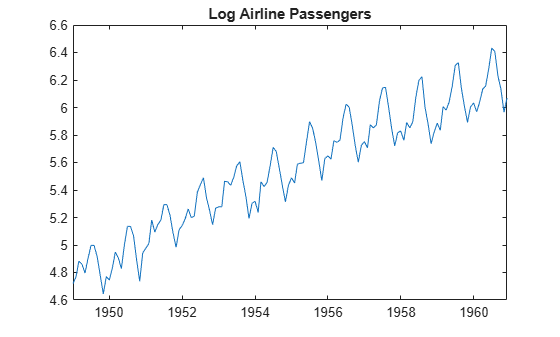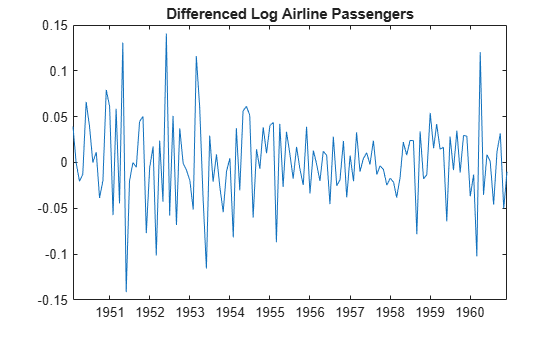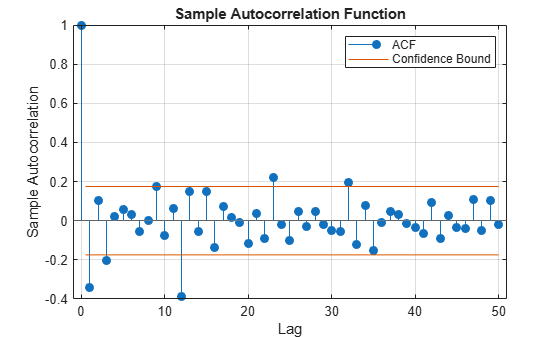# Specify Multiplicative ARIMA Model

This example shows how to specify a seasonal ARIMA model using `arima`. The time series is monthly international airline passenger numbers from 1949 to 1960.

### Load the airline passenger data.

Load the airline data set, and then plot the natural log of the monthly passenger totals.

```load('Data_Airline.mat') y = log(Data); T = length(y); figure plot(dates,y) xlim([1,T]) datetick('x','mmmyy') axis tight title('Log Airline Passengers') ylabel('(thousands)')```The data look nonstationary, with a linear trend and seasonal periodicity.

### Plot the seasonally integrated series.

Calculate the differenced series, $\left(1-L\right)\left(1-{L}^{12}\right){y}_{t}$, where ${y}_{t}$ is the original log-transformed data. Plot the differenced series.

```A1 = LagOp({1,-1},'Lags',[0,1]); A12 = LagOp({1,-1},'Lags',[0,12]); dY = filter(A1*A12,y); figure plot(dY) title('Differenced Log Airline Passengers')```The differenced series appears stationary.

### Plot the sample autocorrelation function (ACF).

```figure autocorr(dY,'NumLags',50)```The sample ACF of the differenced series shows significant autocorrelation at lags that are multiples of 12. There is also potentially significant autocorrelation at smaller lags.

### Specify a seasonal ARIMA model.

Box, Jenkins, and Reinsel suggest the multiplicative seasonal model,

`$\left(1-L\right)\left(1-{L}^{12}\right){y}_{t}=\left(1-{\theta }_{1}L\right)\left(1-{\Theta }_{12}{L}^{12}\right){\epsilon }_{t},$`

for this data set (Box et al., 1994).

Specify this model.

```Mdl = arima('Constant',0,'D',1,'Seasonality',12,... 'MALags',1,'SMALags',12)```
```Mdl = arima with properties: Description: "ARIMA(0,1,1) Model Seasonally Integrated with Seasonal MA(12) (Gaussian Distribution)" Distribution: Name = "Gaussian" P: 13 D: 1 Q: 13 Constant: 0 AR: {} SAR: {} MA: {NaN} at lag  SMA: {NaN} at lag  Seasonality: 12 Beta: [1×0] Variance: NaN ```

The property `P` is equal to `13`, corresponding to the sum of the nonseasonal and seasonal differencing degrees (1 + 12). The property `Q` is also equal to `13`, corresponding to the sum of the degrees of the nonseasonal and seasonal MA polynomials (1 + 12). Parameters that need to be estimated have value `NaN`.

References:

Box, G. E. P., G. M. Jenkins, and G. C. Reinsel. Time Series Analysis: Forecasting and Control. 3rd ed. Englewood Cliffs, NJ: Prentice Hall, 1994.